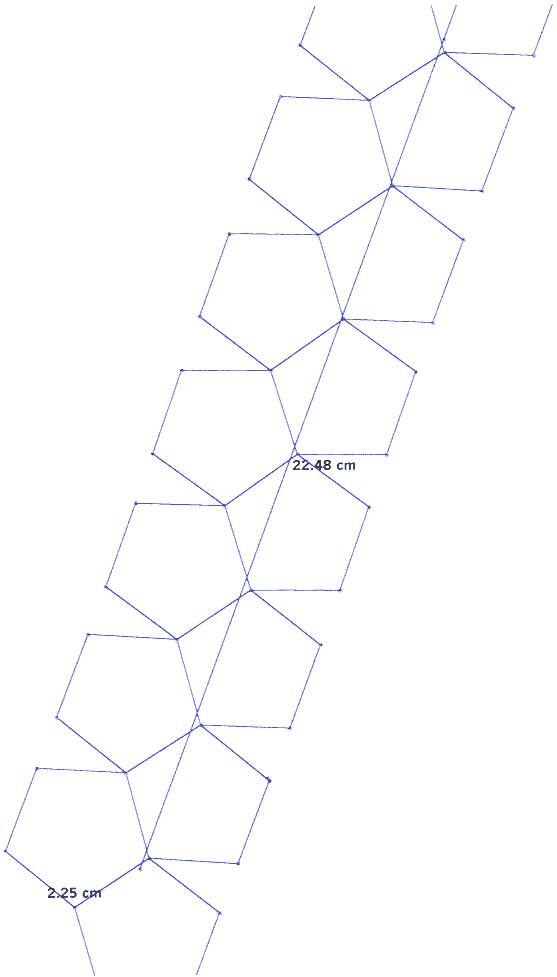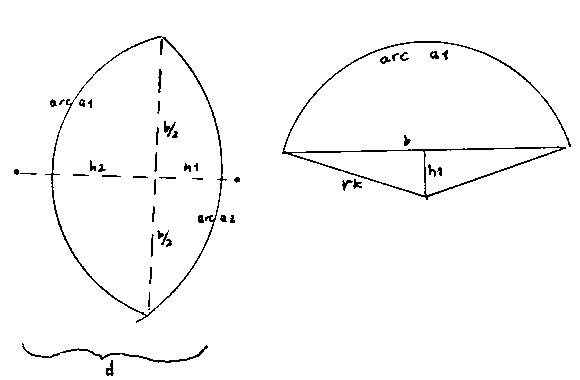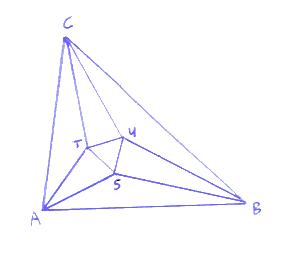Newton's problem

In Crux Mathematicorum I found the following problem, attributed to Sir Isaac Newton:

If 75 cows have in 12 days grazed all the grass in a 60 acre pasture, and 81 cows have in 15 days grazed all the grass in a 72 acre pasture, how many cows can in 18 days graze all the grass in a 96 acre pasture?

My solution runs as follows:

Let E be the amount (in acres) of grass that a cow eats in a day and A the amount of grass that grows in an acre in a day.
From 75*12*E = 60 + 12*60*A and 81*15*E = 72 + 15*72*A we find A = 1/12 and E = 2/15.
Then from x*18*E = 96 + 18*96*A we find x = 100.

mnemonic

For π = 3.14159265358979323846..., I wrote the following mnemonic:

Mum, I fear I might nevermore
be clever, sharp and smart,
although searching further knowledge for
to get decimals will reward.

wallpaper with flowers

The group of symmetry is generated by:
rotations over 60 degrees about any angle of any triangle
and over 120 degrees about the intersection point
of the perpendiculars of any triangle;
reflections in any side of any triangle
and in any perpendicular of any triangle;
translations along any side of any triangle.

The fraction green is: 2*pi/sqrt(3) - 3.

trajectory of a billiard-ball on a triangular table (30-60-90 graden) with mirrorsSqrt(n) in more decimals than the calculator gives

(7 July 1999:)
On my birthday I stand you some little roots: a recipe to have the calculator quickly calculate square roots of natural numbers (which should be not too large) in about twenty signifiacant figures.
The problem is that the calculator gives only ten figures. The solution is: you do one step Newton-Raphson to approach the positive zero of f(x) = x2 - n , with as a starting point the outcome x0 for sqrt(n) which the calculator gives.

As you know, the formula of Newton-Raphson is

x1 = x0 - f(x0)/f '(x0) = x0/2 + n/(2x0) .

So the recipe becomes:
1) Calculate sqrt(n) with the calculator, this is x0
2) Calculate on a piece of paper x0/2 and 2x0
3) Perform the division algorithm you learned on primary school to calculate the first twenty figures of
(n times a billion)/ (2x0 times a billion);
for this, use the following little BASIC program (or a PASCAL variant or ... )
4) Now calculate on a sheet of paper x0/2 + n/(2x0) in twenty figures. This turns out to be a matter of copying and a little attention about the tenth figure.

Enjoy your meal. For ruminants: why is it impossible to approach in this way cubic roots in twenty figures?

10 INPUT A
20 INPUT B
30 M = INT(B/A)
40 PRINT M
50 R = B - M*A
60 B = 10*R
70 GOTO 30

billiard table with five angles

In the magazine Nieuw Archief voor Wiskunde (New Maths Archive) of june 2001 you can find the following open problem:
A billiard table has the form of a regular pentagon with side 1. Lay a billiard-ball anywhere on the table and cue in any direction. Suppose it rolls to a distance 10. What is the maximum number of times it hits a side of the table?

Answer: I did several tries with Cabri, and FOURTEEN turns out to be optimal. Here is an example of such a try with fourteen hits:### On maximal conflict probabilities with grazing goats

On a large planar grass-field two pins are in the ground a distance d apart. To each of both pins a goat is bound with a cord of length rg and one of length rk respectivily (rg > rk), and rg < d < rg+rk (so the goats can touch each other but not the other's pin).You can see in the drawing to the left that the goats can meet each other in a 'domain of conflict' which consists of two circular sectors.
In the drawing to the right you can see how you can calculate the area of such a circular sector:
the area of the 'piece of cake' is a1*rk2/2,
the area of the triangle is h1*b/2 = rk*cos(a1/2)*rk*sin(a1/2) = rk2*sin(a1)/2,
so the area of the circular sector is rk2*(a1 - sin(a1))/2.
So the total area of the domain of conflict is
opptot = rk2*(a1 - sin(a1))/2 + rg2*(a2 - sin(a2))/2.

Given d, rk and rg, you can calculate a1 and a2 with the equalities
(1) (b/2 = ) rk*sin(a1/2) = rg*sin(a2/2), and
(2) d = (h1 + h2 = ) rk*cos(a1/2) + rg*cos(a2/2).
The result is :
sin(a2) = (2*(d2 + rg2 - rk2)/(2*d*rg))*sqrt(1 - ((d2 + rg2 - rk2)/(2*d*rg))2),
and an analogous expression for sin(a1).

The fraction of time that both goats are grazing in the domain of conflict is:
the product of opptot/(pi*rk2) and opptot/(pi*rg2).
The probability of confrontation is directly proportional to this product divided by opptot, so to
(a1 - sin(a1))/(rg2) + (a2 - sin(a2))/(rk2) as well.
In the following little Pascalprogram, we calculate this last expression with
rk=10 and a series of values of rg and d:You can see the results by clicking on results.

It turns out that the probability of confrontation is maximal when both cords have approximately equal length and the distance between the pins is sligthly less than the mean of rg and rk+rg.

A generalisation of the proposition of MorleyGiven an arbitrary triangle ABC. When you draw in each angle the three trisectrices, and you take near each side the intersection point of the two trisectrices that are closest to that side, then the three intersection points are the angular points of a regular triangle (see NIEUWE WISKRANT, sept 2001).
I wondered whether this is true as well if I divide the angle in n equal parts with n>3 instead of three equal parts. If I take near each side the two n-sectrices that are closest to that side, is it true that the three intersection points are the angular points of a regular triangle?
Of course this is true if the original triangle is regular. But in other cases?
I calculated the outcome with vector geometry. Take A(0,0) and B(1,0). Suppose that the angle at A measures a degrees, and at B b degrees (a and b integral and ranging from 1 to 89, so 7921 pairs). For each n from 3 to 10 I calculated with the computer for how many pairs (a,b) the triangle STU is (almost) regular, taking into account rounding errors.
The norm is that each pair out of the three lengths of line segments ST, SU and TU differ less than epsilon. If eps=0.0001 I get for n=3: 7921 pairs, and for n=4 t/m 100: 1. This seems to indicate that it is only true for n>3 if both a and b are 60 degrees.
See the pascal program .
With an adapted version of this program, I derive for n=3: the ratio of areas of the STU and ABC is at most 0.03414828, this maximum occurs when the triangle ABC is regular, and this ratio approaches 0 if the area of ABC approaches 0. Of course, this can be proved in an analytical way. The center of the inscribed circles of ABC and STU do only coincide if ABC is regular.

A nice discontinuous function

Consider the function f from [0,1] to [0,1] that assigns (for example) to 0.325013... 0.11101101111100101110... (substitute for each figure in the original the accessory number of ones and one 0). The function is discontinuous in each point of (0,1) that has only a finite number of decimals unequal to 0, for instance in 0.23 (for this kind of points we choose the representation with the nines to calculate f, so 0.22999999... instead of 0.23).
The function is injective and increasing and the maximum value is
f(1) = f(0.99999...) = 0.111111111011111111101111111110... = 1111111110/9999999999.
notice that x and f(x) are either both rational or both irrational (because the decimal representations are either both repeating or both non-repeating).

A nice new transcendental number

The set of algebraic numbers is enumerable and therefore has measure 0.
So almost all real numbers are transcendental. Nevertheless, it is rather difficult to give other examples of transcendental numbers except algebraic expressions in pi or e.
To begin with, we have the proposition of Liouville, and accessory examples like SUM(k=1,2,3,...: 10^(-k!)) = 0.11000100000000000000000100..
We get another nice example by constructing an enumeration of the algebraic numbers in (0,1), and applying the Cantor diagonal procedure on this enumeration.
I did this with the help of an enumeration of the rational numbers that begins with q1=0, q2=1, q3=-1, q4=2, q5=-2, q6=1/2, ... , and an enumeration of the polynomials with rational coefficients which enumerates (in lexicographical order) first all polynomials with degree 0 (or less) and coefficient q0, then all polynomials with degree 1 (or less) and coefficients q0 and q1, then all polynomials with degree 2 (or less) and coefficients q0, q1 and q2, etc.
If I enumerate the algebraic numbers of (0,1) in order of their occurring as a zero of a polynomial in the list of polynomials mentioned above (zeroes of the same polynomial in order from small to large), and apply to the so-constructed list a1, a2, a3 ... of decimal representations of algebraic numbers the Cantor diagonal procedure, then I find the reuvers transcendental number rtn with n-th decimal equal to an(n)+1(mod 10). This number begins with rtn = 0.71.. (I have not yet calculated more decimals).
Notice that this number is most probably not an algebraic expression in pi or e (because these expressions form a set that is enumerable, so with measure 0).

problem for discussion

When somebody draws a triangle, what is the probability that the triangle is acute-angled?

First solution: choose three times an arbitrary point in the plane. The probability that the third point is thus situated with respect to the other two points, that the triangle formed by these three points is acute-angled, is zero.

Second solution: choose an arbitrary angle alpha between 0 and 180 degrees, and then an arbitrary angle beta between 0 and 180 degrees such that alpha + beta < 180. The probability that the triangle with angles alpha and beta is acute-angled, is 1/2 * 2/3 * 1/2 = 1/6.

diophantine equations related to irreducible polynomials

The irreducibility of the polynomial x3 + 2 over the rationals provides for the unsolvability of the equation x3 + 4y3 -2z3 + 6xyz = 0 for rationals x,y,z (not all zero). This result can be extended to similar results for any irreducible polynomial with rational coefficients.
PROOF: Let I be the ideal (x3+2)Q[x]; the residue class ring Q[X]/I is a field.
For any three rationals a,b,c (not all 0), the residue class a + bx + cx2 + I has a unique inverse A + Bx + Cx2 + I, for which we have:
(a + bx + cx2 + I)(A + Bx + Cx2 + I) = (aA-2bC-2cB) + (aB+bA-2cC)x + (aC+bB+cA)x2 + I = 1 + I.
So for any triple (a,b,c) of rationals (not all 0), the system of linear equations

aA - 2 cB - 2bC = 1
bA + aB - 2cC = 0
cA + bB + aC = 0

has a unique solution (A,B,C).
So the determinant of the coefficient matrix, a3 + 4c3 -2b3 + 6abc, is not 0. This proves our assertion.

When you have three integers a,b,c that are relatively prime and with a+b=c, then it doesn't often occur that each of a,b and c has a large exponent in the prime factor decomposition.
So here is a nice challenge: find a,b,c such that the minimum of the greatest exponents in the prime factor decompositions of a,b,c is as large as possible.
The champion in this field is, as far as I know, a person named Nitaj. He found a=318*23*2269, b=173*29*318, c=210*52*715. So the minimum of the maximal exponents is 8 in his triple. But I guess that he didn't find this result with a simple little pc.
I found a relatively nice result with a simple Pascal program: a=28*3=768, b=7*115=1127357, c=55*192=1128125.
So in my own triple the minimum is 5. Who can find a better result than mine?

random walk

Consider a triangular lattice of points (i,j) with i and j integer, i at most 29, j at least 0 and at most i.
A particle starts at the top (0,0) and takes 60 random steps. What is the probability that it ends up in the bottom row (that is, in a point with i=29)?
I wrote a Pascal program to make a computer simulation. With 32767(=maxint) runs, the frequency of the particle ending up in the bottom row was 0.00003051851.
So I daresay that the probability is about 0.00003.

About a bullet and a cube

Suppose someone shoots a small bullet through a face of a large cube and the bullet leaves the cube through another face. What is the probability that these two faces are opposite faces of the cube?

For this problem I wrote and ran the following Pascal program (using coordinates such that the opposite faces are y=0 and y=1):

program seitz;
const pi=3.1415926536;
var r1,r2,r3,r4,s1,s2,m,n:real;
begin
m:=0; n:=0;
while true do
begin
n:=n+1;
r1:=random; r2:=random; r3:=random; r4:=random;
s1:=r1+cos(r4*pi)/sin(r4*pi); s2:=r2+cos(r3*pi)/(sin(r3*pi)*sin(r4*pi));
if ((0 < s1) and (s1 < 1) and (0 < s2) and (s2 < 1)) then m:=m+1;
writeln(m/n:13:10)
end
end.

The outcome was 0.0745 (rounded).

Integral triangles

Looking for new triangles with integral side lengths a,b,c and vertices with integral coordinates, I took A(0,0), B(c,0), C(p,q), and demanded (p,q,b) and (p-c,q,a) be pythagorean triples.

I wrote and ran variants of a Pascal program with the following pith:

for c:=1 to 20 do for d:=1 to 10 do for u:=1 to 10 do for v:=1 to u do
begin p:=d*(u*u-v*v); q:=2*d*u*v; b:=d*(u*u+v*v); a:=sqrt((p-c)*(p-c)+q*q);
if a=round(a) then writeln(p:10,q:10,a:13:0,b:13:0,c:10) end

I found the expected rectangular triangles, but also unexpected ones like

A(0,0), B(4,0), C(9,12), (a,b,c)=(13,15,4)
A(0,0), B(6,0), C(3,4), (a,b,c)=(5,5,6)
A(0,0), B(14,0), C(5,12), (a,b,c)=(15,13,14)
A(0,0), B(17,0), C(7,24), (a,b,c)=(26,25,17)

When solving a maths problem, it often occurs that we "smell" the solution, like a hound that smells a hare; but we can't catch it at the same time.
And yet, the answer seems so simple afterwards.
That makes me think of the words of Saint Paul: " .. that they might seek God, if perhaps they might grope for Him and find Him, even though He is not far from each one of us."